# MHD Flow and Heat Transfer of a Jeffrey Fluid over a Porous Stretching/Shrinking Sheet with a Convective Boundary Condition

MHD Flow and Heat Transfer of a Jeffrey Fluid over a Porous Stretching/Shrinking Sheet with a Convective Boundary Condition

Dondu Harish Babu Nainaru Tarakaramu Panyam Venkata Satya NarayanaGanganapalli Sarojamma Oluwole Daniel Makinde

Dept. of Mathematics, SreeVidyanikethan Eng. College, Tirupati 517502, A.P, India

Department of Mathematics, SAS, VIT University, Vellore 632014, T.N, India

Department of Applied Mathematics, Sri Padmavati Mahila University, Tirupati 517502, A.P, India

Faculty of Military Science, Stellenbosch University, Private Bag X2, Saldanha 7395, South Africa

Corresponding Author Email:
psatya@vit.ac.in
Page:
885-894
|
DOI:
https://doi.org/10.18280/ijht.390323
23 September 2019
|
Accepted:
10 December 2020
|
Published:
30 June 2021
| Citation

OPEN ACCESS

Abstract:

This work explores the heat transfer flow characteristics of an incompressible non-Newtonian Jeffrey fluid over a stretching/shrinking surface with thermal radiation and heat source. The sheet is linearly stretched in the presence of a transverse magnetic field with convective boundary conditions. Appropriate similarity variables are used to transform the basic governing equations (PDEs) into ODEs. The resulting equations are solved by utilizing MATLAB bvp4c. The impact of distinctive physical parameters and dimensionless numbers on the flow field and heat transfer is analysed graphically. It is noticed that the measure of heat raised with increasing the Biot number and opposite effect with the rise of the suction parameter.

Keywords:

Jeffrey fluid, convective boundary conditions, magneto-hydrodynamics (MHD), thermal radiation, numerical study

1. Introduction

In a real situation, many industrial fluids such as ketchup, pastes, slurries, paint, shampoo, blood, glues, printing inks, food materials, soap and detergent slurries, polymer solutions are non-Newtonian in nature. These fluids are basically non-linear and normally show both viscous and elastic properties. The constitutive equations relating such fluids are intrinsically more sophisticated than conventional Newtonian (Navier-Stokes) fluids. Most non-Newtonian models (Maxwell models, Oldroyd-B models, Walters-B short memory models, Jeffrey model and Eyring-Powell models etc.) involve varying degrees of refinement to the classical momentum conservation equations. Out of these, Jeffrey model is the simplest one rate type non-Newtonian liquids which exhibits shear diminishing attributes, yield pressure and high shear viscosity. Due to its simplicity and variety of applications in science and engineering, many researchers have been attracted. Kothandapani and Srinivas  contemplated the peristaltic flow of a Jeffrey liquid over an asymmetric channel in the occurrence of the magnetic field. Radiative effect on rheological fluid (Jeffrey fluid) over a stretchable cylinder in a 2D flow has been deliberated by Hayat et al. . Khan et al.  presented the influence of third-grade nanofluid flow generated due to sheet stretching. Mishra et al.  implemented a shooting method to analyze the 2D flow of Jeffrey fluid through the stretchable sheet. Narayana et al.  discussed the viscous dissipation effects on Jeffrey fluid flow caused due to a stretchable sheet. More recently, many authors have studied the diverse non-Newtonian fluid flow models with various heat transfer effects [6-10].

The flow analysis of an electrically conducting non-Newtonian liquid over a shrinking/stretching surface plays a vital role in mechanical and engineering applications such as metal spinning, hot rolling and shrinking film for packing of huge products etc. Shrinking surface flow is not quite same as that of stretching sheet flow due to the attraction of fluid towards the origin (slot). Hamid et al.  assumed a time-dependent magnetic field for analyzing the nature of Ohmic heating and viscous dissipation on Williamson fluid flow generated by stretching/shrinking sheet. Yasin et al.  considered the effect of thermal radiation on MHD flow past a shrinking/stretching sheet and solved the model by obtaining the dual solutions. Gangadhar et al.  investigated the effect of Newtonian heating on MHD micropolar nano-liquid in the case of stretching and shrinking surface. They dissected the existence of dual answers for the cases of stretching and shrinking. Jahan et al.  illustrated the influence of nanofluid over a stretching /shrinking sheet. Later, several authors discussed the effect of MHD flow on various fluid models [15-22].

The boundary layer flow with convective boundary conditions and heat transfer has much importance due to their applications in manufacturing and ecological technologies including energy storage, nuclear plants, gas turbines, geothermal reservoirs and rocket propulsion. In view of this, many investigators [23-28] concentrated the heat transfer and mass transfer concepts over various flow geometries. Zeeshan et al.  explored the Couette-Poiseuille flow of viscous nanofluid with convective boundary conditions by utilizing HAM technique. Shehzad et al.  analyzed the 3-D stretched flow of an incompressible Jeffery liquid with convective conditions. Tlili et al.  applied thermodynamics theory to analyze the flow of nanoliquid through a static wedge with convective boundary conditions. The impact of chemical reaction on MHD flow of Jeffery nanoliquid by utilizing the convective boundary conditions was described by Kothandapani and Prakash . The impact of the heat source on Jeffrey liquid over a stretching sheet in the presence of nonlinear energy and concentration has been focused by Narayana and Babu . Recently, Ashraf et al.  and Mishra et al.  discussed the 2D flow of non-Newtonian convective flow with heat source/sink.

Motivated by these facts, a mathematical model on MHD boundary layer flow of a Jeffrey liquid with heat transfer owing to a permeable stretching sheet is proposed. Instead of frequently used uniform surface temperature, a convective boundary condition is applied which makes the analysis unique and further the consequences are accurate and practically valuable. An appropriate similarity transformation is applied to convert the basic governing equations (PDEs) into ODEs which are then solved by utilizing MATLAB bvp4c. The effects of various parameters on the flow field are shown graphically and discussed. It is believed that the present results not only find the applications in realistic engineering but also assist as a complement to the earlier research works.

2. Mathematical Formulation

A steady two-dimensional (x, y) boundary layer flow of an incompressible non-Newtonian Jeffrey liquid over a stretching sheet with thermal radiation and heat source is analysed. In the Cartesian coordinate system, the linear velocity of stretching sheet as $u_{w}(x)=b x$ (where b is a real number) is toward the x-axis and y-axis is opposite to it [see Figure 1]. Also, it is considered that the stretching surface is heated due to convection from a hot liquid at temperature Tf. Additionally, a uniform magnetic field of strength B0 is forced normal to the plate along the y-axis and the induced magnetic field is neglected because the magnetic Reynolds number is very small. The expressions for the Cauchy and the extra stress tensors τ and S1 in a Jeffery non-Newtonian liquid are defined as follows :

$\tau=-p I+S_{1}$    (1)

$S_{1}=\frac{\mu}{1+\lambda}\left[A_{1}+\lambda_{1}\left(\frac{\partial}{\partial t}+V . \nabla\right) A_{1}\right]$    (2)

In which p is the pressure. The Rivlin-Ericksen tensor A1 is defined as $A_{1}=\nabla V+(\nabla V)^{\prime}$, where V is the velocity field vector.

The governing equations of non-Newtonian liquid under the assumption of boundary layer approximations is given by (Ref. [8, 23]).

## 1.png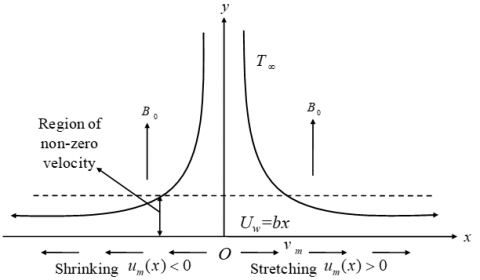Figure 1. Geometry of the problem

$\frac{\partial u}{\partial x}+\frac{\partial v}{\partial y}=0$    (3)

$u \frac{\partial u}{\partial x}+v \frac{\partial u}{\partial y}$

$=\frac{v}{1+\lambda}\left\{\frac{\partial^{2} u}{\partial y^{2}}+\lambda_{1}\left[\begin{array}{c}\frac{\partial u}{\partial y} \frac{\partial^{2} u}{\partial x \partial y}+u \frac{\partial^{3} u}{\partial x \partial y^{2}} \\ -\frac{\partial u}{\partial x} \frac{\partial^{2} u}{\partial y^{2}}+v \frac{\partial^{3} u}{\partial y^{3}}\end{array}\right]\right\}$

$-\frac{v}{K^{*}} u-\frac{\sigma B_{0}^{2}}{\rho} u$    (4)

$u \frac{\partial T}{\partial x}+v \frac{\partial T}{\partial y}$

$=\alpha^{*} \frac{\partial^{2} T}{\partial y^{2}}-\frac{1}{\rho c_{p}} \frac{\partial q_{r}}{\partial y}$

$+\frac{Q_{0}}{\rho c_{p}}\left(T-T_{\infty}\right)$    (5)

The boundary conditions:

$\left.\begin{array}{r}u=u_{w}(x)=b x, v=v_{w}, \\ -k \frac{\partial T}{\partial y}=h\left(T_{f}-T\right) \text { at } \quad y=0 \\ u=0, u^{\prime} \rightarrow 0, T \rightarrow T_{\infty} \\ \text { as } y \rightarrow \infty\end{array}\right\}$    (6)

Utilizing the Rosseland approximation, the radiative heat flux qris defined as :

$q_{r}=-\frac{4 \sigma^{*}}{3 K_{s}} \frac{\partial T^{4}}{\partial y}$    (7)

where, Ks and σ* is the mean absorption coefficient and Stefan-Boltzmann constant respectively. It is assumed that the variation in temperature within the flow is such that T4 can be expanding in Taylor series about T and neglecting higher-order terms beyond the first degree in (T-T), we get:

$T^{4} \approx 4 T_{\infty}^{3} T-3 T_{\infty}^{4}$    (8)

Now, differentiating Eq. (7) w. r. to y and using Eq. (8), we get:

$\frac{\partial q_{r}}{\partial y}=-\frac{16 T_{\infty}^{3} \sigma^{*}}{3 K_{s}} \frac{\partial^{2} T}{\partial y^{2}}$    (9)

Using Eq. (9) in Eq. (5), we obtain:

$u \frac{\partial T}{\partial x}+v \frac{\partial T}{\partial y}$

$=\sigma \frac{\partial^{2} T}{\partial y^{2}}+\frac{1}{\rho c_{p}} \frac{16 T_{\infty}^{3} \sigma^{*}}{3 K_{s}} \frac{\partial^{2} T}{\partial y^{2}}$

$+\frac{Q_{0}}{\rho c_{p}}\left(T-T_{\infty}\right)$    (10)

The following similarity transformations are introduced.

$u=\operatorname{axf}^{\prime}(\eta)$,

$v=-\sqrt{a v} f(\eta), \theta(\eta)$

$=\frac{T-T_{\infty}}{T_{f}-T_{\infty}}, \eta=y \sqrt{\frac{a}{v}}$,

$\psi=x \sqrt{a v} f(\eta)$    (11)

where, the stream function ψ defined as $u=\frac{\partial \psi}{\partial y}, v=-\frac{\partial \psi}{\partial x}$.

Utilizing Eq. (11), the Eqns. (4)-(5) are reduced into the following 3rd and 2nd order ordinary differential equations.

$f^{\prime \prime \prime}+\beta\left(f^{\prime \prime 2}-f f^{\prime \prime \prime}\right)+(1+\lambda)\left(f f^{\prime \prime}-f^{\prime 2}\right)$

$-(1+\lambda)\left(M+\frac{1}{K}\right) f^{\prime}=0$    (12)

$\left(1+\frac{4}{3} R\right) \theta^{\prime \prime}+\operatorname{Pr} f \theta^{\prime}+Q \theta=0$    (13)

and Eq. (6) becomes,

$f=S, f^{\prime}=b / a=\alpha$,

$\theta^{\prime}=-\gamma[1-\theta(0)]$ at $\eta=0$    (14)

$f^{\prime}=0, \quad f^{\prime \prime}(\eta)=0$,

$\theta=0$ as $\eta \rightarrow \infty$    (15)

where, $\beta=\lambda_{1} a$, $K=\frac{v}{a K^{*}}$, $M=\frac{\sigma B_{0}^{2}}{\rho a}$, $P r=\frac{v}{\sigma}$, $R=\frac{4 \sigma^{*} T_{\infty}^{3}}{K_{1} k}$ $Q=\frac{v Q_{0}}{\rho c_{p}}$, $S=-\frac{v_{w}}{\sqrt{a v}}$ (S>0 for suction and S<0 for injection), $\alpha=\frac{b}{a}$ and $\gamma=\frac{h}{k} \sqrt{\frac{v}{a}}$.

The physical quantities of interest are which is defined as $R e_{x}^{\frac{1}{2}} C_{f}=\frac{1}{1+\lambda}\left(f^{\prime \prime}(0)+\beta f^{\prime \prime}(0)\right)$.

The surface drag coefficient (Cf) and the rate of thermal energy (Nu) is defined as:

$C_{f}=\frac{\tau_{w}}{\frac{1}{2} \rho u_{w}^{2}}, \quad N u_{x}=\frac{x\left(q_{w}+q_{r}\right)}{k\left(T_{f}-T_{\infty}\right)}$    (16)

where, $\tau_{w}=\frac{\mu}{1+\lambda}\left\{\left(\frac{\partial u}{\partial y}\right)+\lambda_{1}\left[u \frac{\partial^{2} u}{\partial x \partial y}+u \frac{\partial^{2} v}{\partial x^{2}}+v \frac{\partial^{2} u}{\partial y^{2}}\right]\right\}$ and $q_{w}=-k\left(\frac{\partial T}{\partial y}\right)_{y=0}$, qw is heat transfer from the sheet.

Substituting the value of $\tau_{w}$ and qw into Eq. (16) we get the skin friction and local Nusselt number in dimensionless form as follows:

$c_{f}=2\left(\operatorname{Re}_{x}\right)^{-\frac{1}{2}} \alpha^{-\frac{3}{2}}$

$\cdot\left[\frac{1}{1+\lambda}\left\{\begin{array}{l}f^{\prime \prime}(0)+\beta\left(f^{\prime}(0) f^{\prime \prime}(0)\right. \\ \left.-f(0) f^{\prime \prime}(0)\right)\end{array}\right\}\right]$    (17)

$N u_{x} \operatorname{Re}_{x}^{-1 / 2}=-\left(1+\frac{4}{3} R\right) \theta^{\prime}(0)$    (18)

where, $R e_{x}=\frac{u_{w}(x)}{v}$ is the local Reynolds number.

3. Numerical Procedure

The non-linear ordinary differential Eqns. (12) and (13) with the boundary conditions (14) and (15) have been solved numerically utilizing fourth-order Runge-Kutta method together with shooting technique (see Ref. ). This technique is explained as follows:

1. The higher-order, non-linear differential equations are reduced into a system of first order simultaneous differential equations.
2. The resulting IVP (Initial Value Problem) is solved by utilizing a fourth order Runge-Kutta scheme (see Refs. [38, 39]).
3. Suitable guess values are obtained by using the Newton Raphson method.
4. The calculations have been performed via MATLAB bvp4c.

The iteration process is repeated until the boundary conditions are satisfied.

4. Results and Discussion

The problem of MHD two-dimensional heat transfer flow of a Jeffrey liquid over a shrinking/stretching sheet is studied numerically. The Jeffrey fluid parameters λ and β are chosen in the range of 0≤λ≤2.0, 0≤β≤0.5. The present non-Newtonian model reduced to a viscous model in the absence of Jeffrey fluid parameters λ and β. A parametric study is conducted on Newtonian and Jeffrey fluids, in order to get the pertinent parameters on various flow fields. The velocity, skin-friction coefficient, temperature, and Nusslet number results are analysed through the plotted in Figures 2-13. Table 1, gives the comparison values of Nusslet number with those of Chen  and Grubka and Bobba  in the absence of M, Q, R, λ, β, K and γ. It is noticed that the comparison shows a concurrence with the existing outcomes and thus confirms the exactness of numerical code applied in the current work.

Table 1. Comparison results of $N u_{x} R e_{x}^{-1 / 2}$ for different values of Pr (in the absence of K, γ, β, M, R, Q and λ)

 Pr Chen  Grubka and Bobba  Present 0.01 0.00990 0.00991 0.009000 0.72 0.46315 0.46314 0.463146 1.0 0.58199 0.58199 0.581979 10.0 2.03079 2.30800 2.308008

Table 2, depicts the effect of suction/injection parameter (S), magnetic field (M) and Deborah number (β) on the skin-friction coefficient and Nusselt number along with a variation of Pr. It is seen that the skin friction coefficient decreased with increasing suction parameter (S>0) and β, while the inverse pattern is seen with S<0 and M. Further, an increase in β and M increases the Nusselt Number.

Figure 2 demonstrates the influence of magnetic field parameter M on the velocity profiles against η for both Newtonian and non-Newtonian fluid cases. It is observed that the increase in M reduces the fluid velocity and boundary layer thickness. Physically, an applied magnetic field generates a reverse force (Lorentz force) in the flow field and causes to oppose the movement of liquid. Also, it is noticed that the dimensionless fluid velocity at the sheet has a higher value for a Newtonian fluid (λ=β=0) than to the non-Newtonian fluid (λ, β>0).

Table 2. Numerical Values of $R e_{x}^{\frac{1}{2}} C_{f}$ and $N u_{x} R e_{x}^{-1 / 2}$ for different values of S, M, β and Pr (other parameters are fixed) (i.e. K=2.0; Q=1.0; R=0.4; α=0.3; λ=0.2; γ=1.0)

 S M $\beta$ Pr=1.0 Pr=3.0 $R e_{x}^{\frac{1}{2}} C_{f}$ $\frac{N u_{x}}{\operatorname{Re}_{x}^{1 / 2}}$ $R e_{x}^{\frac{1}{2}} C_{f}$ $\frac{N u_{x}}{\operatorname{Re}_{x}^{1 / 2}}$ 1.0 1.0 0.2 1.45651 0.08211 1.55417 0.09483 0.5 1.25516 0.06838 1.35234 0.08970 0.0 1.02148 0.01778 1.11647 0.05410 -0.5 10.74085 0.25238 11.2166 0.15141 -1.0 5.96083 0.16669 6.21564 0.15203 0.5 0.2 1.94889 0.06830 1.94889 0.08986 0.5 2.11414 0.06808 2.11414 0.08980 1.0 2.36225 0.06775 2.36225 0.08970 1.5 2.58344 0.06747 2.58344 0.08961 0.2 0.1 1.64132 0.06819 1.64132 0.08983 0.2 1.57243 0.06830 1.57243 0.08986 0.3 1.51673 0.06839 1.51673 0.08989 0.4 1.47082 0.06845 1.47082 0.08990

## 2.pngFigure 2. Effect of M on $f^{\prime}(\eta)$

Figures 3(a) and 3(b) illustrate the effect of velocity ratio parameter (α=b/a) on the velocity and temperature distributions. It is noticed that α>0 relates to the sheet stretching while α<0 demonstrate the shrinking sheet respectively. When α = 0 then there is no flow. It is seen that the velocity increases with the increase of α for both the stretching and shrinking cases. Also, it is observed that the rate transport of velocity curve decreases with the increasing distance η normal to the sheet and the velocity vanishes at η=2 from the sheet. Whereas α has a reverse trend in case of the temperature profile.

Figures 4(a) and 4(b) demonstrate the impact of Deborah number β on the velocity and temperature functions for both stretching and shrinking cases. The velocity becomes constant when α=0, decreases for shrinking case and increases for stretching case. It is obvious from the figure that the momentum boundary layer converges quickly for smaller β values. Physically, small β resembles a situation where the material has time to relax (Newtonian nature) and high β relates to non-Newtonian nature. Also, the temperature profile θ(η) reduced with increasing values of β for stretching case.

## 3a.png## 3b.png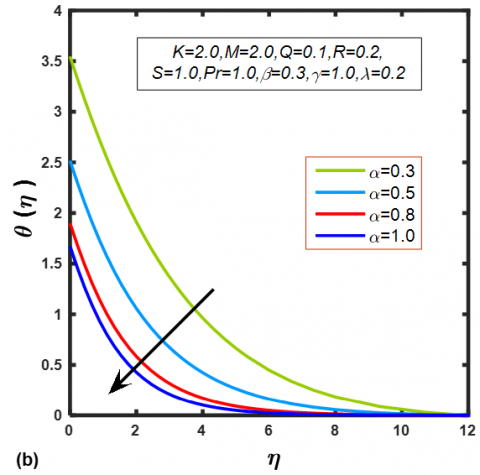Figure 3. Effect of α on (a) f'(η) (b) θ(η)

## 4a.png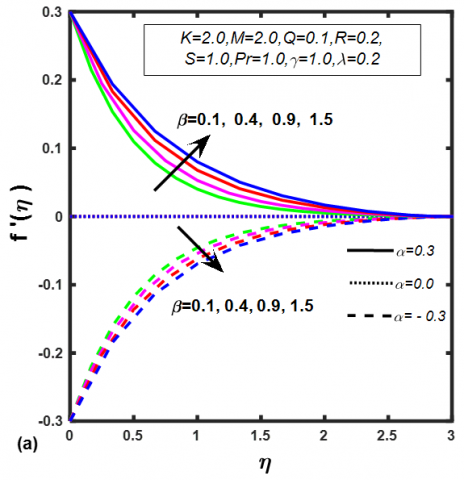## 4b.pngFigure 4. Effect of β on (a) f'(η) (b) θ(η)

Figure 5(a) shows the influence of the ratio of relaxation and retardation times parameter λ on the velocity profiles for stretching and shrinking sheets. It is observed that the velocity decreases with the increase of λ in stretching case and reverse in shrinking case. Physically, an increase in λ means a reduction in fluid retardation time which in turn to stop the hastening of fluid motion. On the other hand, the temperature profile increases with the increase of λ which is obvious from Figure 5(b). Physically, an increase in λ leads to a rise in relaxation time and diminish in retardation time, due to this higher temperature and thicker thermal boundary layer.

The effect of permeability parameter K on the velocity profiles is shown in Figure 6. It is observed that in the case of stretching sheet (α>0) the velocity increases with the increase of K and β values. These results are similar to those obtained in Ref. . It is also noticed from the figure that the velocity boundary layer converges quickly for small values of β.

Figures 7(a), 7(b) and 7(c) respectively show the influence of suction/injection parameter S on the velocity and temperature profiles. It is obvious from the figures that f'(η) and θ(η) is found to a decrease with the increase of S>0. On the other hand, inverse behaviour is seen in the injection case (S<0).

## 5a.png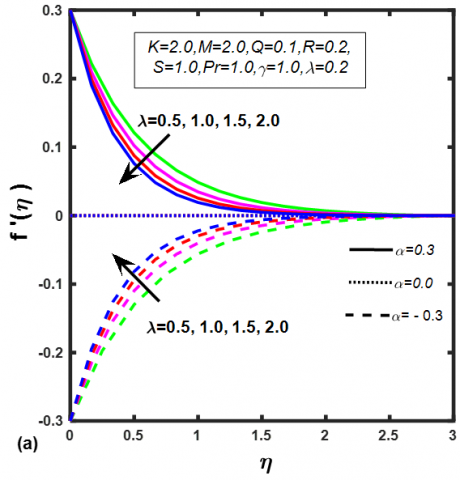## 5b.pngFigure 5. Effect of λ on (a) f'(η) (b) θ(η)

## 6.png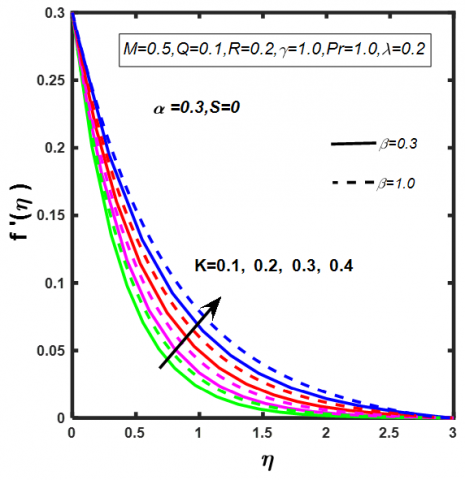Figure 6. Effect of K on f'(η)

## 7a.png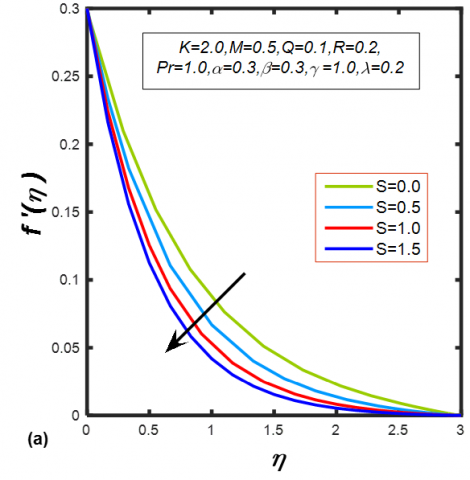## 7b.png## 7c.png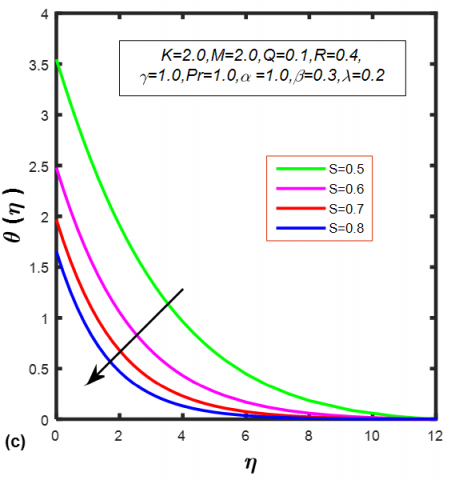Figure 7. Effect of (a) S≥0 on f'(η) (b) S < 0 on f'(η) (c) S>0 on θ(η)

The influence of Biot number γ on θ(η) is plotted in Figure 8. It is evident that the θ(η) increase with the rise in γ for both stretching and shrinking cases. In general, γ=0 represents the no convective heat exchange from the surface of the sheet to the cold fluid which is far away from the sheet. For this situation, it should be understood that the surface is totally insulated. Further, it is observed that the temperature is greater in the case of shrinking when compared to the stretching sheet. These outcomes obviously reinforced from the physical perspective.

The effect of thermal radiation parameter R on the dimensionless temperature profile is offered in Figure 9. It is inspected that the fluid temperature increases by an increase in R values. This is because of the fact that as R increases; the mean absorption coefficient Ks diminishes. Hence, the divergence of radiation heat flux increases. As a result, the rate of radiative heat transfer into the liquid rises.

Figure 10 represents the temperature profile for different values of heat source/sink parameter (Q>0 and Q<0). It is observed that the boundary layer generates the energy, which causes the temperature profile to increase with increasing values of the heat source (Q>0) whereas reverse effects are seen in the heat sink (Q<0) case. This is due to the fact that the rise of Q in the boundary layer makes energy which roots the θ(η) increase. From this, it is concluded that in the engineering and industrial heat transfer applications the heat sink is well appropriate for real cooling of the stretching sheet. It is also important to note that θ(η) decreases with increasing values of the suction parameter (S>0).

## 8.png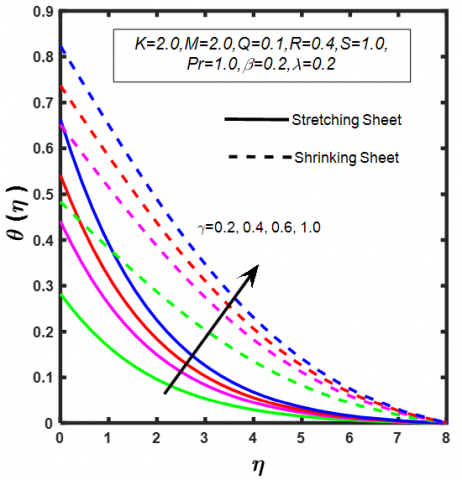Figure 8. Effect of γ on θ(η)

## 9.pngFigure 9. Effect of R on θ(η)

Figure 11(a) portrays the attributes of skin friction coefficient concerning λ and β for stretching and shrinking cases. It is found that the skin-friction reduce in case of stretching sheet with rising β and reverse a trend in the case of a shrinking sheet. Figure 11(b) shows the influence of β on Nusselt number for stretching/shrinking sheet. The Nusselt number raised in terms of increase in β for stretching sheet, while it is reversed in case of the shrinking sheet.

Figures 12(a) and 12(b) describe the changes in Nusselt number against Prandtl number Pr for various values of Q and R respectively. It is perceived from the graphs that Nusselt number decline with the augmented values of Q and rises for Pr. The heat transfer coefficient for viscous fluid is more than that of Jeffrey fluid. In addition, the opposite trend is noticed with the rise in R.

## 10.png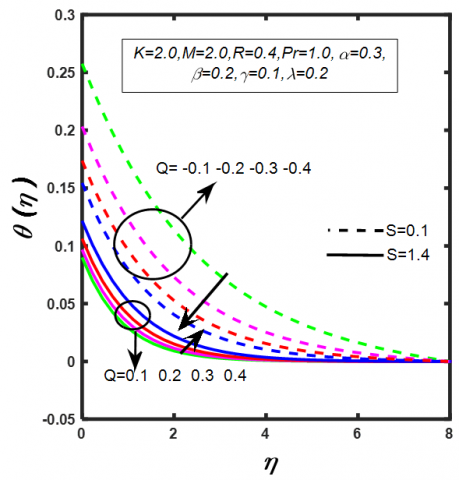Figure 10. Effect of Q on θ(η)

## 11a.png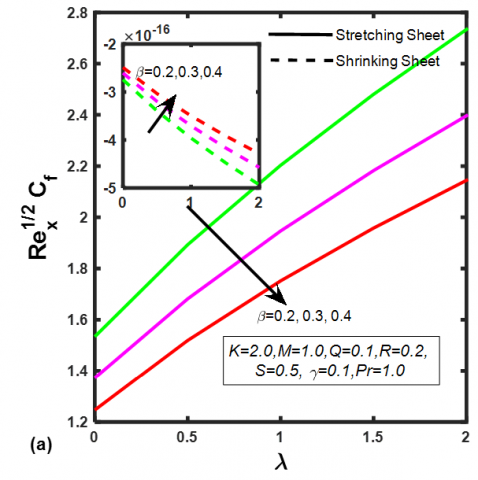## 11b.png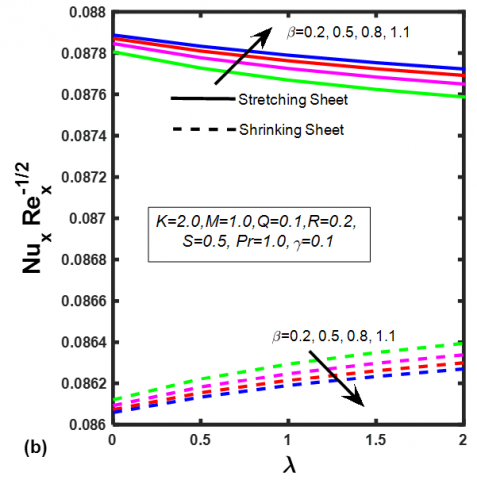Figure 11. Effect of λ against β on (a) $C f_{x} R e_{x}^{1 / 2}$ (b) $N u_{x} R e_{x}^{-1 / 2}$

## 12a.png## 12b.pngFigure 12. Effect of Pr against (a) Q on $N u_{x} R e_{x}^{-1 / 2}$ (b) R on $N u_{x} \operatorname{Re}_{x}^{-1 / 2}$

## 13.pngFigure 13. Comparison of the velocity distribution for various values of α between present and Hayat et al.  work

In order to check the accuracy of the present results, we have analyzed the outcomes of the velocity distribution for different numbers of α in Figure 13 with those reported by Hayat et al.  in the absence of M and K. This correlation shows an incredible arrangement.

5. Conclusions

Two-dimensional boundary layer flow of MHD heat transfer of a Jeffrey fluid with thermal radiation and heat source/sink is discussed numerically in this paper. The main points of this analysis are given below:

1. The velocity of Jeffery fluid increases with rising stretching ratio parameter.
2. Temperature distributions have the same behaviour for different values of γ and R.
3. The variation of S on the temperature distributions is qualitatively the same when γ>0.
4. f’(η) enhanced for augmented values of K.
5. The velocity profile f’(η)was declined with raising values of M for both Newtonian and non-Newtonian cases.
6. The skin friction coefficient shows reverse nature in stretching and shrinking sheet cases for diverse values of β.
7. The rate of heat transfer exhibits the same behaviour in Newtonian and non-Newtonian cases for different values of Q.
Nomenclature
 a Constant A1 Rivlin-Ericksen tensor B0 magnetic induction [T] b real number cp specific heat at constant pressure [J/kg/K] F dimensional stream function f' dimensionless velocity h convective heat transfer K permeability of the porous medium k thermal conductivity of fluid [W/m/k] Ks Rosseland mean absorption coefficient M magnetic field R radiation parameter Nu Nusselt number Pr Prandtl number Q heat source/sink parameter qr radiative heat flux[W/m] qw surface heat flux Rex local Reynolds number S suction/injection parameter T fluid temperature (K) Tf convective fluid temperature below the moving sheet Tw wall temperature on a sheet at y = 0 (K) T∞ temperature far away from the wall (i) u, v velocity components in x-,y-directions, respectively [m/s] uw Velocity of stretching sheet [ms-1] vw suction velocity across a stretching sheet x the distance along wall [m] y distance normal to the wall [m] Greek symbols α ratio parameter β Deborah number η similarity variable γ Biot number $\lambda$ ratio of relaxation and retardation times λ1 retardation time [s] $\mu$ dynamic viscosity [Pa/s] υ kinematic viscosity [m2s-1] ρ fluid density [kg/m] σ electric conductivity[sm-1] σ* Stefan-Boltzmann constant [Wm-2K-4] θ non-dimensional temperature τ Cauchy stress tensor τw shear stress along stretching sheet ψ steam function Subscripts w sheet surface ∞ Infinity Superscript ' differentiation with respect to η
References

 Kothandapani, M., Srinivas, S. (2008). Peristaltic transport of a Jeffrey fluid under the effect of magnetic field in an asymmetric channel. International Journal of Non-Linear Mechanics, 43(9): 915-924. https://doi.org/10.1016/j.ijnonlinmec.2008.06.009

 Hayat, T., Asad, S., Alsaedi, A., Alsaadi, F.E. (2015). Radiative flow of Jeffrey fluid through a convectively heated stretching cylinder. Journal of Mechanics, 31(1): 69-78. https://doi.org/ 10.1017 /jmech.2014.49

 Khan, W.A., Culham, J.R., Makinde, O.D. (2015). Combined heat and mass transfer of third‐grade nanofluids over a convectively‐heated stretching permeable surface. The Canadian Journal of Chemical Engineering, 93(10): 1880-1888. https://doi.org/10.1002/cjce.22283

 Mishra, A.K., Senapati, N. Mishra, S.R., Bhattacharjee, S. (2018). Chemical reaction effect on forced convection flow of a Jeffrey fluid over a stretching sheet: a numerical study. Diffusion Foundations, 16: 109-119. https://doi.org/10.4028/www.scientific.net/DF.16.109

 Narayana, P.V.S., Babu, D.H., Babu, M.S. (2019). Numerical study of a Jeffrey fluid over a porous stretching sheet with heat source/sink. International Journal of Fluid Mechanics Research, 46(2). https://doi.org/10.1615/InterJFluidMechRes.2018025030

 Gangadhar, K., Sobhana Babu, P.R., Makinde, O.D. (2018). Spectral relaxation method for Powell-Eyring fluid flow past a radially stretching heated disk surface in a porous medium. Defect and Diffusion Forum, 387: 575-586. https://doi.org/10.4028/www.scientific.net/DDF.387.575

 Sarojamma, G., Vijaya Lakshmi, R., Sreelakshmi, K., Vajravelu, K. (2020). Dual stratification effects on double-diffusive convective heat and mass transfer of sheet-driven micropolar fluid flow. J. King Saud University - Sci., 32(1): 366-376. https://doi.org/10.1016/j.jksus.2018.05.027

 Hayat, T., Sadia, A., Qasim, M., Awatif A.H. (2012). Boundary layer flow of a Jeffrey fluid with convective boundary conditions. Int. J. Numer. Meth. Fluids, 69: 1350-1362. https://doi.org/10.1002/fld.2642

 Morteza, B., Nader, A. (2020). Study of laminar convection heat transfer of non-Newtonian nanofluids in a tube under constant heat flux using new power-law relation. Int. J. Heat and Technology, 38(2): 507-515. https://doi.org/10.18280/ijht.38022

 Sarojamma, G., Vijaya Lakshmi, R., Satya Narayana, P. V., Animasaun, I.L. (2020). Exploration of the significance of autocatalytic chemical reaction and Cattaneo-Christov heat flux on the dynamics of a micropolar fluid. Journal of Applied and Computational Mechanics, 6(1): 77-89. https://doi.org/10.22055/jacm.2019.28742.1501

 Hamid, A., Khan, M., Hafeez, A. (2018). Unsteady stagnation-point flow of Williamson fluid generated by stretching/shrinking sheet with Ohmic heating. International Journal of Heat and Mass Transfer, 126: 933-940. https://doi.org/10.1016/j.ijheatmasstransfer.2018.05.076

 Yasin, M.H.M., Ishak, A., Pop, I. (2016). MHD heat and mass transfer flow over a permeable stretching/shrinking sheet with radiation effect. Journal of Magnetism and Magnetic Materials, 407: 235-240. https://doi.org/10.1016/j.jmmm. 2016.01.087

 Gangadhar, K., Kannan, T., Jayalakshmi, P. (2017). Magnetohydrodynamic micropolar nanofluid past a permeable stretching/shrinking sheet with Newtonian heating. Journal of the Brazilian Society of Mechanical Sciences and Engineering, 39(11): 4379-4391. https://doi.org/10.1007/s40430-017-0765-1

 Jahan, S., Sakidin, H., Nazar, R., Pop, I. (2018). Analysis of heat transfer in nanofluid past a convectively heated permeable stretching/shrinking sheet with regression and stability analyses. Results in Physics, 10: 395-405. https://doi.org/10.1016/j.rinp.2018.06.021

 Ellahi, R., Bhatti, M.M., Riaz, A., Sheikholeslami, M. (2014). Effects of magnetohydrodynamics on peristaltic flow of Jeffrey fluid in a rectangular duct through a porous medium. Journal of Porous Media, 17(2). https://doi.org/10.1615/JPorMedia.v17.i2.50

 Aleem, M., Asjad, M.I., Ahmadian, A., Salimi, M., Ferrara, M. (2020). Heat transfer analysis of channel flow of MHD Jeffrey fluid subject to generalized boundary conditions. The European Physical Journal Plus, 135(1): 1-15. https://doi.org/10.1140/epjp/s13360-019-00071-6

 Babu, D.H., Narayana, P.S. (2016). Joule heating effects on MHD mixed convection of a Jeffrey fluid over a stretching sheet with power law heat flux: A numerical study. Journal of Magnetism and Magnetic Materials, 412: 185-193. https://doi.org/10.1016/j.jmmm.2016.04.011

 Malik, M.Y., Makinde, O.D. (2018). Parabolic curve fitting study subject to Joule heating in MHD thermally stratified mixed convection stagnation point flow of Eyring-Powell fluid induced by an inclined cylindrical surface. Journal of King Saud University-Science, 30(4): 440-449. https://doi.org/10.1016/j.jksus.2017.02.003

 Kumaraswamy Naidu, K., Harish Babu, D., Harinath Reddy, S., Satya Narayana, P.V. (2020). Radiation and partial slip effects on MHD Jeffrey nanofluid containing gyrotactic microorganisms over a stretching surface. Journal of Thermal Science and Engineering Applications, pp. 1-28. https://doi.org/10.1115/1.4048213

 Makinde, O.D. (2012). Heat and mass transfer by MHD mixed convection stagnation point flow toward a vertical plate embedded in a highly porous medium with radiation and internal heat generation. Meccanica, 47(5): 1173-1184. https://doi.org/10.1007/s11012-011-9502-5

 Babu, D.H., Ajmath, K.A., Venkateswarlu, B., Narayana, P.V. (2019). Thermal radiation and heat source effects on MHD non-Newtonian nanofluid flow over a stretching sheet. Journal of Nanofluids, 8(5): 1085-1092. https://doi.org/10.1166/jon.2019.1666

 Seini, I.Y., Makinde, D.O. (2014). Boundary layer flow near stagnation-points on a vertical surface with slip in the presence of transverse magnetic field. International Journal of Numerical Methods for Heat & Fluid Flow, 24(3): 643-653. https://doi.org/10.1108/HFF-04-2012-0094

 Ishak, A. (2010). Similarity solutions for flow and heat transfer over a permeable surface with convective boundary condition. Applied Mathematics and Computation, 217(2): 837-842. https://doi.org/10.1016/j.amc.2010.06.026

 Makinde, O.D., Aziz, A. (2011). Boundary layer flow of a nanofluid past a stretching sheet with a convective boundary condition. International Journal of Thermal Sciences, 50(7): 1326-1332. https://doi.org/10.1016/j.ijthermalsci.2011.02.019

 Mhamed, T., Mohamed, N.B. (2020) Deep investigation on natural convection flow of a couple stress fluid with nanoparticles in an MHD vertical porous channel with convective boundary conditions. Int. J. Heat and Technology, 38(2): 487-498. https://doi.org/10.18280/ijht. 380226

 Makinde, O.D. (2011). Similarity solution for natural convection from a moving vertical plate with internal heat generation and a convective boundary condition. Thermal Science, 15(1): 137-143. https://doi.org/10.2298/TSCI11S1137M

 Rundora, L., Makinde, O.D. (2015). Effects of Navier slip on unsteady flow of a reactive variable viscosity non-Newtonian fluid through a porous saturated medium with asymmetric convective boundary conditions. Journal of Hydrodynamics, 27(6): 934-944. https://doi.org/10.1016/S1001-6058(15)60556-X

 Shateyi, S., Makinde, O.D. (2013). Hydromagnetic stagnation-point flow towards a radially stretching convectively heated disk. Mathematical Problems in Engineering. https://doi.org/10.1155/2013/616947

 Zeeshan, A., Shehzad, N., Ellahi, R. (2018). Analysis of activation energy in Couette-Poiseuille flow of nanofluid in the presence of chemical reaction and convective boundary conditions. Results in Physics, 8: 502-512. https://doi.org/10.1016/j.rinp.2017.12.024

 Shehzad, S.A., Alsaedi, A., Hayat, T. (2012). Three-dimensional flow of Jeffery fluid with convective surface boundary conditions. International Journal of Heat and Mass Transfer, 55(15-16): 3971-3976. https://doi.org/10.1016/j.ijheatmasstransfer.2012.03.027

 Tlili, I., Hamadneh, N.N., Khan, W.A. (2019). Thermodynamic analysis of MHD heat and mass transfer of nanofluids past a static wedge with Navier slip and convective boundary conditions. Arabian Journal for Science and Engineering, 44(2), 1255-1267. https://doi.org/10.1007/s13369-018-3471-0

 Kothandapani, M., Prakash, J. (2016). Convective boundary conditions effect on peristaltic flow of a MHD Jeffery nanofluid. Applied Nanoscience, 6(3): 323-335. https://doi.org/10.1007/s13204-015-0431-9

 Narayana, P.S., Babu, D.H. (2016). Numerical study of MHD heat and mass transfer of a Jeffrey fluid over a stretching sheet with chemical reaction and thermal radiation. Journal of the Taiwan Institute of Chemical Engineers, 59: 18-25. https://doi.org/10.1016/j.jtice.2015.07.014

 Ashraf, M.B., Alsaedi, A., Hayat, T., Shehzad, S.A. (2017). Convective heat and mass transfer in three-dimensional mixed convection flow of viscoelastic fluid in presence of chemical reaction and heat source/sink. Computational Mathematics and Mathematical Physics, 57(6): 1066-1079. https://doi.org/10.1134/S0965542517060021

 Mishra, S.R., Khan, I., Al-Mdallal, Q.M., Asifa, T. (2018). Free convective micropolar fluid flow and heat transfer over a shrinking sheet with heat source. Case Studies in Thermal Engineering, 11: 113-119. https://doi.org/10.1016/j.csite.2018.01.005

 Brewster, M.Q. (1972). Thermal Radiation Transfer Properties. John Wiley & Sons: New York, USA.

 Nachtsheim, P.R., Swigert, P. (1965). Satisfaction of the asymptotic boundary conditions in the numerical solution of the system of nonlinear equations of boundary layer type. NASA, Washigton.

 Jain, M.K. (2003). Numerical methods for scientific and engineering computation. New Age International.

 Na, T.Y. (1979). Computational Methods in Engineering Boundary Value Problems, 145 (New York: Academic).

 Chen, C.H. (1998). Laminar mixed convection adjacent to vertical, continuously stretching sheets. Heat and Mass Transfer, 33(5-6): 471-476. https://doi.org/10.1007/s002310050217

 Grubka, L.J., Bobba, K.M. (1985). Heat transfer characteristics of a continuous stretching surface with variable temperature. ASME J. Heat Transfer, 107(1): 248-250. https://doi.org/10.1115/1.3247387